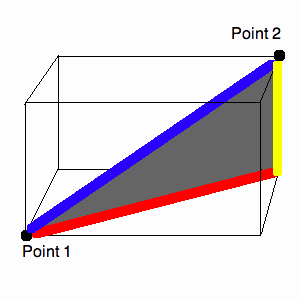# Euclidean Distance

When people speak of "Euclidean distance" they are usually speaking about distances computed in the Cartesian plane or in Cartesian three-dimensional space. In this module you will discover how to compute the distance between two points in either type of space given only their coordinates.

## Distance in the Plane

Before we delve into the whole issue, let's look at a couple of examples.

Problem 1: What is the distance between the points (5, 0) and (-3, 0) in the Cartesian plane? What about between the points (28, 0) and (127, 0)?

Problem 2: What is the distance between the points (8, 1) and (12, 1)?

Problem 3: Can you generalize your results to two arbitrary points on the same horizontal line? That is, if I gave you two points (x1, y) and (x2, y), could you give me the distance between them?

Problem 4: Now let's think about two points on the same vertical line. What is the distance between the points (3, 12) and (3, -5)?

Problem 5: Can you generalize your result to two arbitrary points on the same vertical line?

Now that we have some basic formulas and understanding of distance in the place, we are ready to investigate the distance between any two points in the plane. Before we come up with a general formula, let's do a specific example.

Problem 6: Consider the points (1, 2) and (5, -1) in the plane. Find a right triangle the has these points as vertices at the acute angles. How long are its legs? Using the Pythagorean theorem, find the length of the hypotenuse. How far apart are the two original points?

Problem 7: Repeat the previous problem, but with two arbitrary points in the plane, say (x1, y1) and (x2, y2).

Now we have a formula for distance in the plane!

## Distance in Cartesian Three-Dimensional Space

The work that you did on finding distances in the plane is integral to getting a formula to find distances in three-dimensional space. Let's start out by generalizing your formulas for points on the same horizontal and vertical lines from before. Throughout, I will refer to the first coordinate of a three-dimensional triple as the x-coordinate, the second as the y-coordinate, and the third as the z-coordinate.

Problem 8: What is the distance between the points (1, 2, 3) and (1, 2, -3)? What about (1, 2, 3) and (1, 8, 3)? What about (1, 2, 3) and (10, 2, 3)?

Problem 9: Can you generalize your answers in the previous problem to get formulas for the distance between two points whose coordinates only differ in one place?

Problem 10: Now let's consider an arbitrary two points that have the same third coordinate, that is, two points that lie in the same xy-plane. Can you put them on a right triangle that lies in the same plane? Using the Pythagorean theorem, can you find the distance between them?

Problem 11: Now, given two points in three dimensional space, consider first the one with the least z-coordinate. What is the distance between this point and the point lying on the same xy-plane, directly beneath the second point? That is, given
Point 1 = (x1, y1, z1)
and
Point 2 = (x2, y2, z2), where z1z2, what is the distance between (x1, y1, z1), and (x2, y2, z1)?

Problem 12: Consider the diagram below. It depicts one possible arrangement of points that corresponds to that in problem 11. You found the length of the blue line in problem 11. You found the length of the yellow line in problem 9. Can you use this information, and the Pythagorean theorem to determine the length of the blue line?The formula you found in the last problem is the formula for the distance between two arbitrary points in three-dimensional space!